# The HPGENSELECT Procedure

### Exponential Family Distributions

Many of the probability distributions that the HPGENSELECT procedure fits are members of an exponential family of distributions, which have probability distributions that are expressed as follows for some functions b and c that determine the specific distribution:For fixed, this is a one-parameter exponential family of distributions. The response variable can be discrete or continuous, so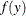represents either a probability mass function or a probability density function. A more useful parameterization of generalized linear models is by the mean and variance of the distribution: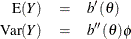In generalized linear models, the meanof the response distribution is related to linear regression parameters through a link function,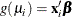for the ith observation, where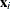is a fixed known vector of explanatory variables andis a vector of regression parameters. The HPGENSELECT procedure parameterizes models in terms of the regression parametersand either the dispersion parameteror a parameter that is related to, depending on the model. For exponential family models, the distribution variance is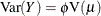where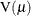is a variance function that depends only on.

The zero-inflated models and the multinomial models are not exponential family models, but they are closely related models that are useful and are included in the HPGENSELECT procedure.